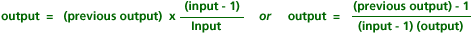# Linear Functions and Slope Part D: Putting It Together (35 minutes)

## Session 5, Part D

Linear functions have come up in many situations so far in this session. In this section, we’ll consolidate some of our ideas about linear functions and look for connections between them. Note 14

Below are several input/output tables. For each table:
Note 15

• Find a closed form rule for taking an input and finding the correct output
• Find a recursive rule for going from one output to the next
• Graph the pairs of numbers in the table
• Determine if the rule describes a linear function or not. If it is a linear function, find the slope.

### Problems

Problem D1

 Input Output 1 0 2 1 3 2 4 3 5 4

Problem D2

 Input Output 1 1 2 4 3 9 4 16 5 25

Tip: The recursive rule here includes n, the input number.

Problem D3

 Input Output 1 3 2 5 3 7 4 9 5 11

Problem D4

 Input Output 1 9 2 8 3 7 4 6 5 5

Problem D5

 Input Output 1 5 2 10 3 15 4 20 5 25

Problem D6

 Input Output 1 1 2 1/2 3 1/3 4 1/4 5 1/5

Problem D7

 Input Output 1 -7 2 -7 3 -7 4 -7 5 -7

Problem D8

What are the characteristics of a linear function? How can you tell that a function is linear if you are given:

• a closed form rule for a function?
• a recursive rule for a function?
• a description of a situation?
• a table?
• a graph?

### Notes

Note 14

The point of this section is to make connections between the different situations in which we’ve seen linear functions.

Note 15

Take 15-20 minutes to work on Problems D1-D7. Then think about the connections in the different representations, paying particular attention to instances where linear functions are different from other kinds of functions.

In Problem D6, the recursive rule for the function y = 1/x can be quite challenging. The easiest way to describe it is to use your input in the rule.Some people consider a rule recursive only if it truly depends on previous outputs, with no reference to the input.

In summary:

• Closed forms for linear functions look like y = ax + b, where a and b are some numbers, x is the independent variable, and y is the dependent variable.
• Recursive rules for linear functions add a constant value from one output to the next. This constant is the same as the value of a in the formula y = ax + b.
• Graphs of linear functions look like lines. The slopes of the lines are the same as the difference between successive outputs, and the same as the value of a in the y = ax + b formula.

Groups:
Discuss the above statements.

### Solutions

Problem D1

The following solutions refer to the input variable as “x” and the output variable as “y.”

The closed-form rule is y = x – 1.

The recursive rule is yn = yn-1 + 1, since the outputs grow by 1 each time.

This is a linear function, according to its graph, and the slope is 1.

Problem D2

The closed-form rule is y = x2.
The recursive rule is harder to formulate for this one: it is yn = yn-1 + (2n – 1). The key here is finding the pattern in the differences between each term. This is not a linear function.

Problem D3

The closed-form rule is y = 2x + 1.
The recursive rule is yn = yn-1 + 2. Outputs grow by 2 each time.
This is a linear function, and the slope is 2.

Problem D4

The closed-form rule is y = -x + 10, or y = 10 – x (both are the same).
The recursive rule is yn = yn-1 – 1. Outputs drop by 1 each time.
This is a linear function, and the slope is -1.

Problem D5

The closed-form rule is y = 5x.
The recursive rule is yn = yn-1 + 5. Outputs grow by 5 each time.
This is a linear function, and the slope is 5.

Problem D6

The closed-form rule is y = 1 / x.
The recursive rule is very difficult. Two possible answers are 1 / yn = 1 / yn-1 + 1, and yn= yn-1 + 1 / (n)(n-1).
This is not a linear function. Notice that the rate of change is not constant.

Problem D7

The closed-form rule is y = -7.
The recursive rule is yn = yn-1, because every term is the same as the last.
This is a linear function, according to the graph, and the slope is 0 (which means it is a horizontal line).

Problem D8

If there is a closed-form rule for a function, and the function is linear, it will be in the form y = Mx + B, where M and B can be any real number — positive, negative, or 0. Note Problems D5 and D7, in which one of the two values is 0.

If there is a recursive rule given, it should be in the form yn = yn-1 + M, where M is the slope of the line.

If a situation is described, it should involve a constant rate of change, such as a constant speed of a car, the constant slope of a ramp, or the constant price of gasoline per gallon.

If a table is given, the rate of change (change in output, divided by change in input) should always be the same number. If inputs are a sequence of numbers (like 1, 2, 3, 4, 5), the outputs should also form a sequence (3, 5, 7, 9, 11; 5, 10, 15, 20, 25).

If a graph is given, it should be a straight line (a linear function).